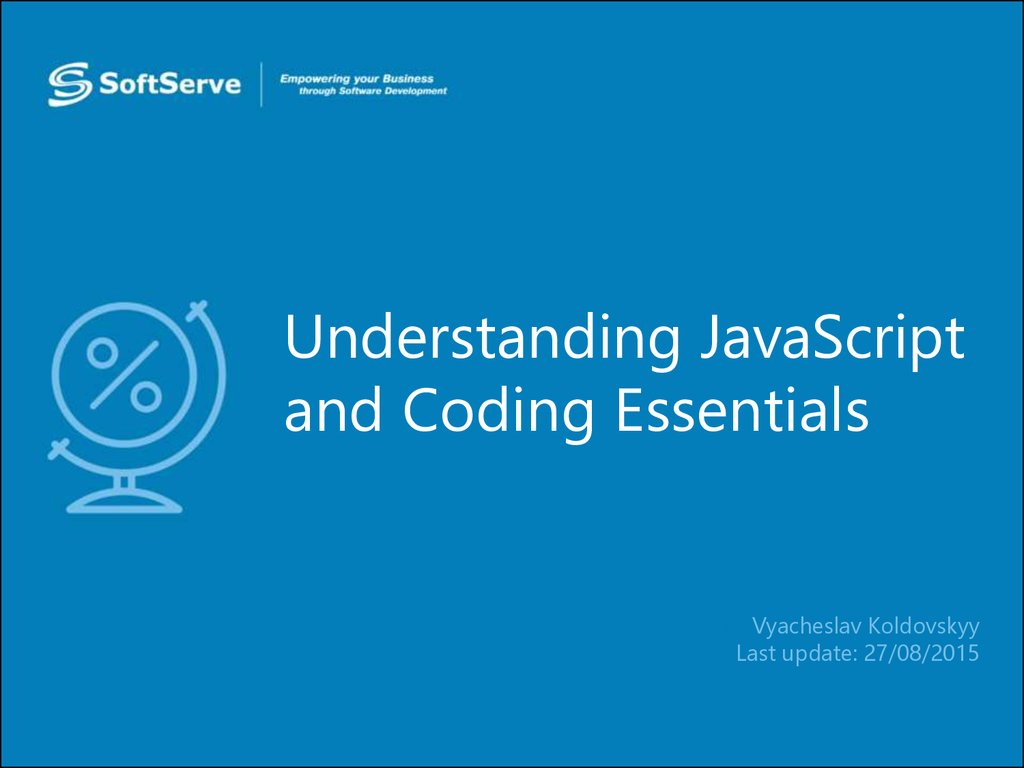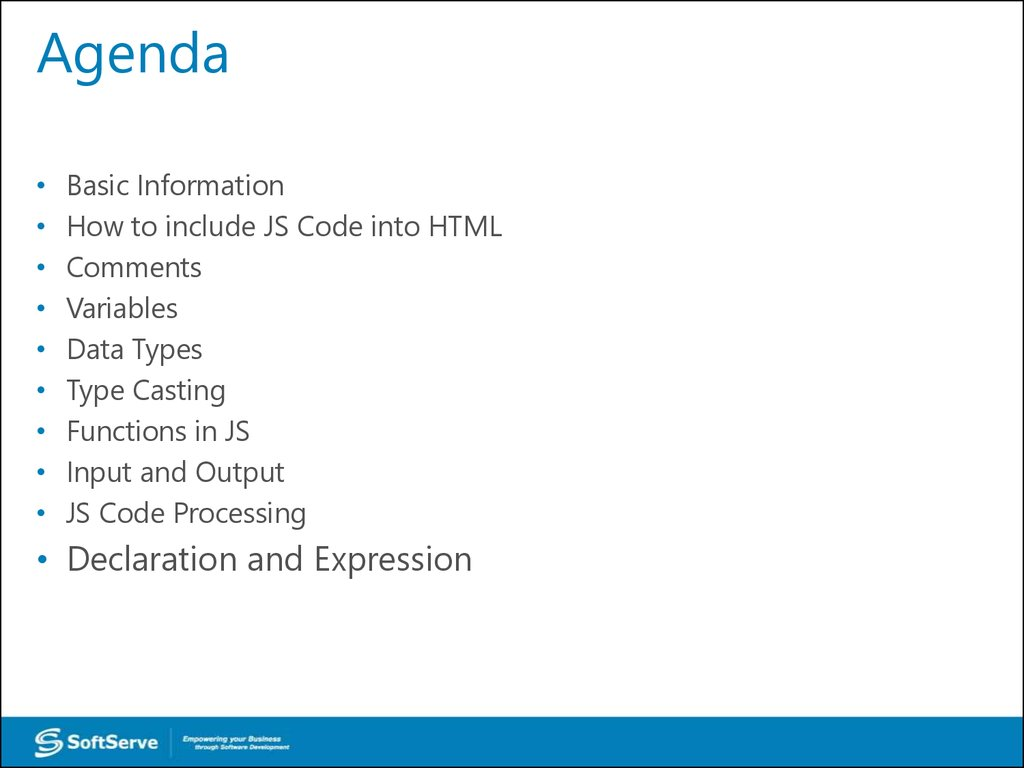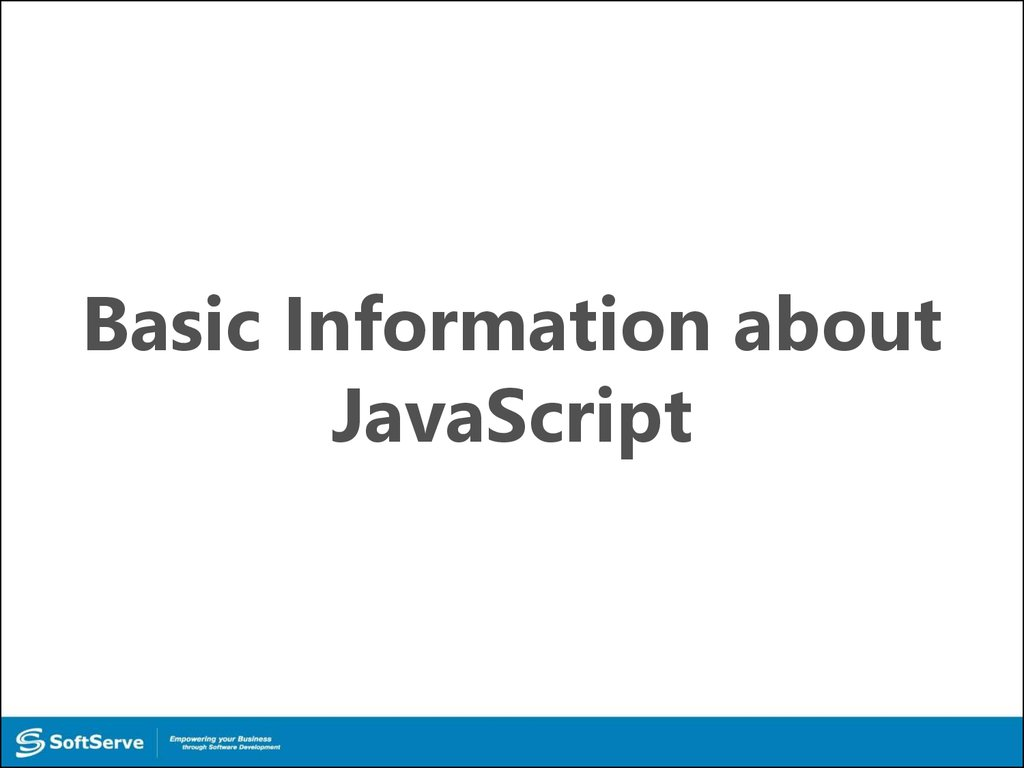# 06-Understanding JavaScript and Coding Essentials

## 1. Understanding JavaScript and Coding Essentials

• Vyacheslav Koldovskyy
Last update: 27/08/2015

## 2. Agenda

Basic Information
How to include JS Code into HTML
Variables
Data Types
Type Casting
Functions in JS
Input and Output
JS Code Processing
• Declaration and Expression

JavaScript

## 4. Basic information

JavaScript - dynamic computer programming
language.
It is most commonly used as part of web browsers,
whose implementations allow client-side to interact with
the user, control the browser and asynchronously
communicate with server-side.
JavaScript syntax was influenced by C.

## 5. Basic information

JS take many names and naming conventions from Java,
but the two languages are otherwise unrelated and have
very different semantics. 
JavaScript is a prototype-based scripting language with
dynamic typing. 
JS supported object-oriented, imperative and functional
programming styles. 

## 6.

How to include JS Code
into HTML

## 7. Including of JavaScript

Exist three ways to include script into
HTML page:
• Inline in HTML 
• Inside tag <script>
• In separate file 


## 8. Inline including

<button onclick = “f();”>…. </button>
Unfortunately, this is the worst solution.
Holistic code will be broken into smaller parts.
Such parts are difficult to test and maintain. :(

## 9. Inside tag <script>

Inside tag <script>
<script>
f() ;
</script>
Sometimes it makes sense.
But in the general case, page size will be increased
and, as a result, its performance reduced.

## 10. In separate file

<script src = “somewhere.js”></script>
This is the best way.
Code is holistic. It’s easy to test and maintain.
In addition, separated files are cached by the browser.
This improves performance. :)

## 11.

Comments - part of the program text which will be
ignored by language interpreter 
The /* characters, followed by any sequence of
characters (including new lines), followed by
the */ characters. 
The // characters, followed by any sequence of
characters, but only in current line. Therefore, it is
commonly called a "single-line comment." 

Variables

## 14. Variables

Variable – symbolic name associated with a value
and whose associated value may be changed. 
Declaration – process of variable's specifying.
Usually declaration consist of defining: type, name
and default value of variable. 
A process in which a variable is set to its first value is
called initialization. 

## 15. Declaration and initialization

var – special keyword for declaration of variables
In JavaScript
var variable;
variable = 10;
//declaration
//initialization

Or quickly
var variable = 10;



## 16. Global and local

JavaScript has two types of variables:
global - exist in memory and is available at all times
of the program. In JS it's a variables of page. 
local - exist in memory and is available only in block
when variable is defined. In JS it's defined in function
variables. 

Data Types

## 18. Data types

JavaScript have 6 base data types:
• Number – scalar type for integer and real digits
• Boolean – scalar type for logical values
• String – special type for work with text information
• Undefined – special type for uninitialized variables
• Null – special type for "cleaning" of variables
• Object – complex type for service and user needs

## 19. Number, Boolean and String

var n = 10; or var n = Number(10);
//number values for example: -1, 10, 3.14, Nan, Infinity
var b = true; or var b = Boolean(true);
//bollean values: true and false
var s = “text”; or var s = String(“text”);
//string values for example: “”, “text”, ‘text’




## 20. Null and Undefined

var n = null;
//null variables can have only null value

var u;
// created and uninitialized
And Object type… but it will be reviewed in future :)

Type Casting

## 22. Type casting

Exist two types of casting:
var a, b, c;
a = 10;
b = true;
c = a + b;

Explicit
Implicit

var a, b, c;
a = 10;
b = true;
c = a + Number(b);
But both ways given c =11 as a result!


## 23. Type casting

Base rules of typing casting:




All scalar types try to convert itself to largest scalar
type: Boolean to Number, Number to String.
If Boolean converted to String it at first converted to
Number and after them Number to String.
In mathematical operations (excluding +) String
should be converted to Number.
Null and Undefined converted to String as “null” and
“undefined”, and to Number as a 0 and NaN

Functions in JS

## 25. Basic Information

In mathematics:
Function is a relation between a set of inputs and a set

of permissible outputs.
y = f(x)

In classical programming
Function is a named part of a code that performs a

distinct service.

## 26. Example

var i, base, power, result;

base = 2; power = 2; result = 1; 
for(i = 0; i < power; i++) { 
result *= base;
}
console.log(result); 
base = 3; power = 4; result = 1;
for(i = 0; i < power; i++) {
result *= base;
}
console.log(result);


## 27. Declaration of function

function is a special keyword for creation of function

in JavaScript.
function name () {
body;
}


## 28. Example

var i, base, power, result;
base = 2; power = 2; result = 1;
for(i = 0; i < power; i++) {
result *= base;
}
console.log(result);
base = 3; power = 4; result = 1;
for(i = 0; i < power; i++) {
result *= base;
}
console.log(result);

## 29. Example

function pow () {
result = 1;
for (var i = 0; i < power; i++) {
result *= base;
}
}

## 30. Function call

Call - operation for execution of function.
( ) – operator for this action.


Usually function can be called by name.


## 31. Example

var base, power, result;
base = 2; power = 2;
pow();
console.log(result);
base = 3; power = 4;
pow();
console.log(result);
function pow () {
result = 1;
for(var i = 0; i < power; i++) {
result *= base;
}
}

Input and Output

## 33. Input and Output

function name (a, b) {
return a + b;
}

* you can return one value only

* return always interrupts the execution.

* place your return at the end of a function


## 34. Example

function pow () {
result = 1;
for (var i = 0, i < power; i++) {
result *= base;
}
}

## 35. Example

function pow (base, power) {
var result = 1;
for (var i = 0, i < power; i++) {
result *= base;
}
return result;
}

## 36. Example

var out;
out = pow(2, 2);
console.log(out);
out = pow(3, 4);
console.log(out);
function pow (base, power) {
var result = 1;
for(var i = 0; i < power; i++) {
result *= base;
}
return result;
}

## 37.

JS Code Processing

## 38. Code processing

var a = 10;
test();
function test () {
a = 30;
var b = 40;
}
var b = 20;
console.log(a, b);

## 39. Code processing

1.
var a = 10;
test();
function test () {
a = 30;
var b = 40;
}
var b = 20;
console.log(a, b);

## 40. Code processing

2.
1.
3.
var a = 10;
test();
function test () {
a = 30;
var b = 40;
}
var b = 20;
console.log(a, b);

## 41. Code processing

2.
4.
1.
5.
3.
6.
var a = 10;
test();
function test () {
a = 30;
var b = 40;
}
var b = 20;
console.log(a, b);

## 42. Code processing

2.
4.
1.
5.
3.
6.
var a = 10;
test();
function test () {
5.2
a = 30;
var b = 40; 5.1
}
var b = 20;
console.log(a, b);

Declaration and
Expression

## 44. Declaration and Expression

function name () {
body;
}
var name = function () {
body;
};



Functions in JavaScript are Objects.
As a result, functions are accessible by
reference.


Functions can be used as a parameter in other

function.
References to functions can be saved in any
other variable.